## How to Calculate and Solve for Standing Bubble Point Parameter | The Calculator Encyclopedia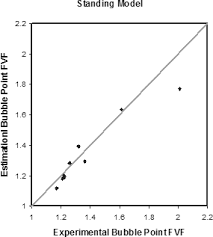The image above represents standing bubble point parameter.

To compute for the standing bubble point parameter, two essential parameters are needed and these parameters are API Gravity (°API) and Temperature (°Rankine) (T).

The formula for calculating standing bubble point parameter:

a = [0.00091(T – 460)] – [0.0125(°API)]

Where:

a = Standing Bubble Point Parameter, a
°API = API Gravity
T = Temperature (°Rankine)

Let’s solve an example;
Find the standing bubble point parameter when API Gravity is 32 and the temperature is 146.

This implies that:

°API = API Gravity = 32
T = Temperature (°Rankine) = 146

a = [0.00091(T – 460)] – [0.0125(°API)]
a = [0.00091(146 – 460)] – [0.0125(32)]
a = [0.00091(-314)] – [0.0125(32)]
a = [-0.28574] – [0.0125(32)]
a = [-0.28574] – [0.4]
a = -0.68574

Therefore, the standing bubble point parameter, a is -0.68574.

## How to Calculate and Solve for Standing Bubble Point | The Calculator Encyclopedia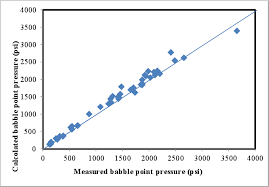The image above represents standing bubble point.

To compute for the standing bubble point, three essential parameters are needed and these parameters are Specific Gravity of Solution Gas (γg), Bubble Point Pressure (Pb) and Standing Bubble Point Parameter (a).

The formula for calculating the standing bubble point:

Rs = 18.2[((Pb / γg)0.83 x 10a) – 1.4]

Where:

Rs = Standing Bubble Point
γg = Specific Gravity of Solution Gas
Pb = Bubble Point Pressure
a = Standing Bubble Point Parameter

Let’s solve an example;
Find the standing bubble point with a specific gravity of solution gas of 24, bubble point pressure of 70 and standing bubble point parameter of 45.

This implies that;

γg = Specific Gravity of Solution Gas = 24
Pb = Bubble Point Pressure = 70
a = Standing Bubble Point Parameter = 45

Rs = 18.2[((Pb / γg)0.83 x 10a) – 1.4]
Rs = 18.2[((70 / 24)0.83 x 1045) – 1.4]
Rs = 18.2[((2.916)0.83 x 1045) – 1.4]
Rs = 18.2[(2.43 x 1045) – 1.4]
Rs = 18.2[2.431e+45 – 1.4]
Rs = 18.2[2.431e+45]
Rs = 4.425e+46

Therefore, the standing bubble point is 4.425e+46.

## How to Calculate and Solve for MBAL Gas Solubility | The Calculator Encyclopedia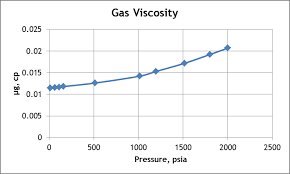The image above represents the MBAL gas solubility.

To compute for the MBAL gas solubility, four essential parameters are needed and these parameters are Specific Gravity of Solution Gas (γg), Specific Gravity of Stock Tank Oil (γo), Oil FVF (Bo) and Oil Density (ρo).

The formula for calculating MBAL gas solubility:

Rs = Boρo – 62.4γo / 0.0136γg

Where:

Rs = MBAL Gas Solubility
γg = Specific Gravity of Solution Gas
γo = Specific Gravity of Stock Tank Oil
Bo = Oil FVF
ρo = Oil Density

Let’s solve an example;
Given that specific gravity of solution gas is 9, specific gravity of stock tank oil is 13, oil FVF is 19 and oil density is 27. Find the MBAL gas solubility?

This implies that;

γg = Specific Gravity of Solution Gas = 9
γo = Specific Gravity of Stock Tank Oil = 13
Bo = Oil FVF = 19
ρo = Oil Density = 27

Rs = Boρo – 62.4γo / 0.0136γg
Rs = 19 x 27 – 62.4 x 13 / 0.0136 x 9
Rs = 513 – 811.19 / 0.1224
Rs = -298.19 / 0.1224
Rs = -2436.27

Therefore, the MBAL gas solubility is -2436.27.

## How to Calculate and Solve for Petrosky-Farshad Gas Solubility Parameter | The Calculator Encyclopedia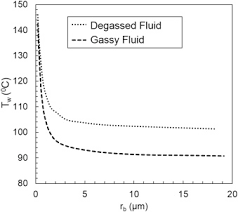The image above represents petrosky-farshad gas solubility parameter.

To compute for the petrosky-farshad gas solubility parameter, two essential parameters are needed and these parameters are API Gravity (°API) and temperature (°Rankine) (T).

The formula for calculating the petrosky-farshad gas solubility parameter:

Rs = [7.716(10-4) (°API)1.541] – [4.561(10-5) (T -460)1.3911]

where:

Rs = Petrosky-Farshad Gas Solubility Parameter, x
°API = API Gravity
T = Temperature (°Rankine)

Let’s solve an example;
Find the petrosky-farshad gas solubility parameter with an API Gravity of 11 and a temperature of 90.

This implies that;

°API = API Gravity = 11
T = Temperature (°Rankine) = 90

Rs = [7.716(10-4) (°API)1.541] – [4.561(10-5) (T -460)1.3911]
Rs = [7.716(10-4) (11)1.541] – [4.561(10-5) (90 – 460)1.3911]
Rs = [7.716(10-4) (11)1.541] – [4.561(10-5) (-370)1.3911]
Rs = [7.716(10-4) (11)1.541] – [4.561(10-5) (NaN)]
Rs = [7.716(10-4) (40.2518)] – [4.561(10-5) (NaN)]
Rs = [0.03105] – [4.561(10-5) (NaN)]
Rs = 0.03105 – NaN
Rs = NaN

Therefore, the Petrosky-Farshad gas solubility parameter, x is NaN.

## How to Calculate and Solve for Petrosky-Farshad Gas Solubility in a Fluid | The Calculator Encyclopedia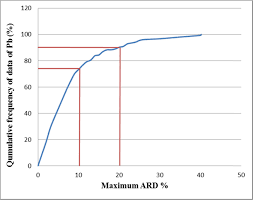The image above represents petrosky-farshad gas solubility.

To compute for the petrosky-farshad gas solubility, three essential parameters are needed and these parameters are gas specific gravity (γg), petrosky-farshad gas solubility parameter (x) and pressure (P).

The formula for calculating the petrosky-farshad gas solubility:

Rs = [(P/112.727 + 12.340)γg0.8439 10x]1.7384

Where:

γg = Gas Specific Gravity
x = Petrosky-Farshad Gas Solubility Parameter
P = Pressure

Let’s solve an example;
Find the petrosky-farshad gas solubility when the gas specific gravity is 16, the petrosky-farshad gas solubility parameter is 22 and the pressure is 80.

This implies that;

γg = Gas Specific Gravity = 16
x = Petrosky-Farshad Gas Solubility Parameter = 22
P = Pressure = 80

Rs = [(P/112.727 + 12.340)γg0.8439 10x]1.7384
Rs = [(80/112.727 + 12.340)160.8439 x 1022]1.9384
Rs = [(0.709 + 12.340)160.8439 x 1022]1.9384
Rs = [(13.049)160.8439 x 1022]1.9384
Rs = [(13.049)10.379 x 1022]1.9384
Rs = [1.354e+24]1.9384
Rs = 8.925e+41

Therefore, the Petrosky-Farshad gas solubility is 8.925e+41.

## How to Calculate and Solve for Marhoun’s Gas Solubility in a Fluid | The Calculator Encyclopedia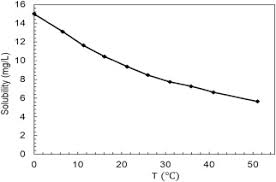The image above represents marhoun’s gas solubility.

To compute for the marhoun’s gas solubility, four essential parameters are needed and these parameters are Gas Specific Gravity (γg), Temperature (°Rankine) (T), Pressure (P) and Stock Tank Oil Gravity (γo).

The formula for calculating the marhoun’s gas solubility:

Rs = [a γgb γoc Td P]e

Where:

Rs = Marhoun’s Gas Solubility
a = 185.843208
b = 1.877840
c = -3.1437
d = -1.32657
e = 1.398441
γg = Gas Specific Gravity
T = Temperature (°Rankine)
P = Pressure
γo = Stock Tank Oil Gravity

Let’s solve an example;
Find the marhoun’s gas solubility when the gas specific gravity is 14, temperature is 80, pressure is 40 and the stock tank oil gravity is 24.

This implies that;

γg = Gas Specific Gravity = 14
T = Temperature (°Rankine) = 80
P = Pressure = 40
γo = Stock Tank Oil Gravity = 24

Rs = [a γgb γoc Td P]e
Rs = [185.843208 x 141.87784 x 24-3.1437 x 80-1.32657 x 40]1.398441
Rs = [185.843208 x 141.98 x 24-3.1437 x 80-1.32657 x 40]1.398441
Rs = [185.843208 x 141.98 x 0.0000458 x 80-1.32657 x 40]1.398441
Rs = [185.843208 x 141.98 x 0.0000458 x 0.00298 x 40]1.398441
Rs = [0.1445]1.398441
Rs = 0.0668

Therefore, the marhoun’s gas solubility is 0.0668.

## How to Calculate and Solve for Glass Gas Solubility Parameter in a Fluid | Nickzom Calculator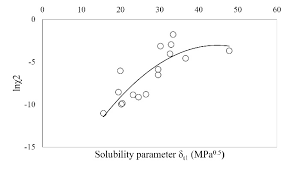The image above represents glass gas solubility parameter.

To compute for the glass gas solubility parameter, one essential parameter is needed and the parameter is Pressure (P).

The formula for calculating the glass gas solubility parameter:

x = 2.8869 – [14.1811 – 3.3093logP]0.5

Where:

x = Glass Gas Solubility Parameter, x
P = Pressure

Let’s solve an example;
Find the glass gas solubility parameter when the pressure is 62.

This implies that;

x = Glass Gas Solubility Parameter, x = 62

x = 2.8869 – [14.1811 – 3.3093logP]0.5
x = 2.8869 – [14.1811 – 3.3093log(62)]0.5
x = 2.8869 – [14.1811 – 3.3093(1.79)]0.5
x = 2.8869 – [14.1811 – 5.93]0.5
x = 2.8869 – [8.249]0.5
x = 2.8869 – 2.87
x = 0.0146

Therefore, the Glass Gas Solubility Parameter is 0.0146.

## How to Calculate and Solve for Mean Bubble Point in a Fluid | The Calculator Encyclopedia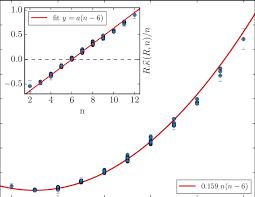The image above represents mean bubble point.

To compute for the mean bubble point, one essential parameter is needed and the parameter is Glass gas solubility parameter (x).

The formula for calculating mean bubble point:

Pb* = 10x

Where:

Pb* = Mean Bubble Point
x = Glass Gas Solubility Parameter

Let’s solve an example;
Given that the glass gas solubility parameter is 12. Find the mean bubble point?

This implies that;

x = Glass Gas Solubility Parameter = 12

Pb* = 10x
Pb* = 1012
Pb* = 1000000000000

Therefore, the Mean bubble point is 1000000000000.

## How to Calculate and Solve for Glass Gas Solubility in a Fluid | The Calculator Encyclopedia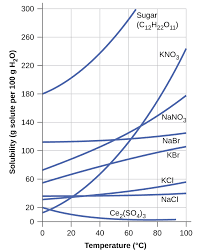The image above represents glass gas solubility.

To compute for the glass gas solubility, four essential parameters are needed and these parameters are API Gravity (°API), Temperature (°Rankine) (T), Mean Bubble Point (Pb*) and Gas Gravity at Actual Separator Psep and Tsepg).

The formula for calculating the glass gas solubility:

γgs = γg[((°API)0.989 / (T – 460)0.172)Pb*]1.2255

Where:

γgs = Glass Gas Solubility
°API = API Gravity
T = Temperature (°Rankine)
Pb* = Mean Bubble Point
γg = Gas Gravity at Actual Separator Psep and Tsep

Let’s solve an example;
Find the glass gas solubility when the API Gravity is 15, the temperature is 30, the mean bubble point is 40 and the gas gravity at actual separator is 54.

This implies that;

°API = API Gravity = 15
T = Temperature (°Rankine) = 30
Pb* = Mean Bubble Point = 40
γg = Gas Gravity at Actual Separator Psep and Tsep = 54

γgs = γg[((°API)0.989 / (T – 460)0.172)Pb*]1.2255
γgs = 54[((15)0.989 / (30 – 460)0.172)40]1.2255
γgs = 54[((15)0.989 / (-430)0.172)40]1.2255
γgs = 54[((15)0.989 / (NaN))40]1.2255
γgs = 54[(14.5 / NaN)40]1.2255
γgs = 54[(NaN)40]1.2255
γgs = 54[NaN]1.2255
γgs = 54[NaN]
γgs = NaN

Therefore, the glass gas solubility is NaN.

## How to Calculate and Solve for Separator Gas Gravity in a Fluid | The Calculator Encyclopedia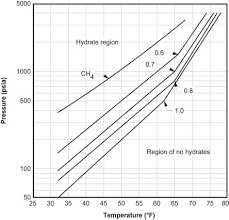The image above represents separator gas gravity.

To compute for the separator gas gravity, four essential parameters are needed and these parameters are API Gravity (°API), Actual Separator Temperature (°Rankine) (Tsep), Actual Separator Pressure (Psep) and Gas Gravity at Actual Separator Psep and Tsepg).

The formula for calculating the separator gas gravity:

γgs = γg[1 + 5.912(10-5)(°API)(Tsep – 460)log(Psep / 114.7)]

Where:

γgs = Separator Gas Gravity
°API = API Gravity
Tsep = Actual Separator Temperature (°Rankine)
Psep = Actual Separator Pressure
γg = Gas Gravity at Actual Separator Psep and Tsep

Let’s solve an example;
Find the separator gas gravity with an API Gravity of 21, actual separator temperature of 18, actual separator pressure of 14 and gas gravity at actual separator of 32.

This implies that;

°API = API Gravity = 21
Tsep = Actual Separator Temperature (°Rankine) = 18
Psep = Actual Separator Pressure = 14
γg = Gas Gravity at Actual Separator Psep and Tsep = 32

γgs = γg[1 + 5.912(10-5)(°API)(Tsep – 460)log(Psep / 114.7)]
γgs = 32[1 + 5.912(10-5)(21)(18 – 460)log(14 / 114.7)]
γgs = 32 [1 + 5.912(10-5)(21)(18 – 460) log(0.1220)]
γgs = 32 [1 + 5.912(10-5)(21)(-442) log(0.1220)]
γgs = 32 [1 + 5.912(10-5)(21) (-442) (-0.9134)]
γgs = 32 [1 + 0.501]
γgs = 32 [1.501]
γgs = 48.039

Therefore, the separator gas gravity is 48.039.